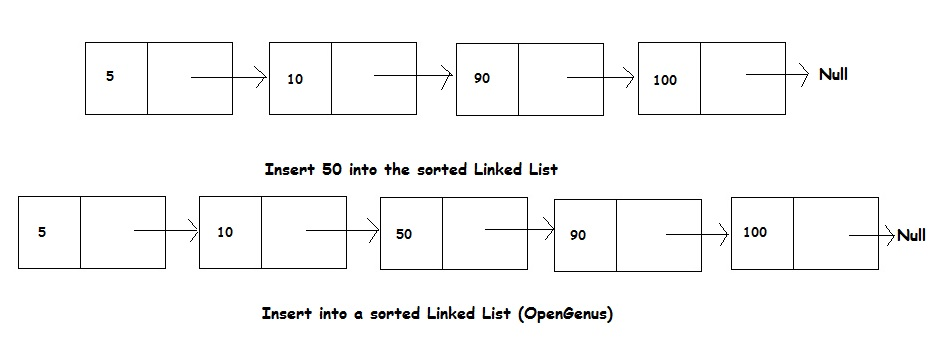×

Search anything:

# Insert element in a sorted Linked ListGet this book -> Problems on Array: For Interviews and Competitive Programming

Reading time: 15 minutes | Coding time: 20 minutes

In this session, we will explore how to insert an element in a sorted Linked List such that the modified Linked List is sorted as well.### Pseudocode

Let input linked list is sorted in increasing order.

STEP 1) If Linked list is empty then make the node as head and return it.

STEP 2) If value of the node to be inserted is smaller than value of head node, then insert the node at start and make it head.

STEP 3) Find the appropriate node after which the input node is to be inserted. To find the appropriate node start from head, keep moving until we reach a node whose value is greater than the input node. The node just before the node is the appropriate node.

STEP 4) Insert the node after the appropriate node found in step 3.

``````
void sortedInsert(E e)
{
Node current = first;
/* Special case for head node */
if (current == null || current.data >= e)
{
}
else
{
while (current.next != null && current.next.data < new_node.data)
current = current.next;
final Node<E> newNode = new Node<>(current, e, current.next);
}
}
``````

• Java

### Java

``````
import java.util.*;
{
private static class Node<E>
{
E item;
Node<E> next;
Node<E> prev;
Node(Node<E> prev, E element, Node<E> next)
{
this.item = element;
this.prev = prev;
this.next = next;
}
}
transient int size = 0;
transient Node<E> first;
transient Node<E> last;
// Creates an empty list
// Sorted Insert in a Linked List
void sortedInsert(E e)
{
Node current = first;
/* Special case for head node */
if (current == null || current.data >= e)
{
}
else
{
while (current.next != null && current.next.data < new_node.data)
current = current.next;
final Node<E> newNode = new Node<>(current, e, current.next);
}
}
// Return Node at index "index" O(N) time
public Node<E> node( int index)
{
if(index < (size >> 1))
{
Node<E> x = first;
for(int i=0;i<index; ++i)
x = x.next;
return x;
}
else
{
Node<E> x = last;
for(int i=size-1; i>index; --i)
x = x.prev;
return x;
}
}
// Print all elements in the LinkedList
public void printList()
{
Node<E> x = first;
for(int i=0;i<size; ++i)
{
System.out.println(x.item);
x = x.next;
}
}
{
final Node<E> front = first;
final Node<E> newNode = new Node<>(null, e, front);
first = newNode;
if( front == null)
last = newNode;
else
front.prev = newNode;
++ size;
}
{
final Node<E> l = last;
final Node<E> newNode = new Node<>(l, e, null);
last = newNode;
if(l == null)
first = newNode;
else
l.next = newNode;
++ size;
}
// Inserts a node before a non null node succ
private void LinkBefore(E e, Node<E> succ)
{
Node<E> before = succ.prev;
Node<E> newNode = new Node<E>(before, e, succ);
succ.prev = newNode;
if(before == null)
{
first = newNode;
}
else
{
before.next = newNode;
}
++ size;
}
{
final Node<E> next = f.next;
first = next;
final E element = f.item;
f.item = null;
f.next = null;
if(next == null)
last = null;
else
next.prev = null;
-- size;
return element;
}
{
final E element = l.item;
final Node<E> newLast = l.prev;
l.prev = null;
l.item = null;
last = newLast;
if(newLast == null)
first = null;
else
newLast.next = null;
-- size;
return element;
}
{
final E element = n.item;
final Node<E> before = n.prev;
final Node<E> next = n.next;
if( before == null )
else if(next == null)
else
{
n.item = null;
n.next = null;
n.prev = null;
before.next = next;
next.prev = before;
-- size;
}
return element;
}
{
}
{
}
{
}
}
{
public static void main()
{
Node<Integer> nn = ll.node(3);
ll.printList();
}
}
``````

### Complexity

• Worst case time complexity: `Θ(N)`
• Average case time complexity: `Θ(N)`
• Best case time complexity: `Θ(1)`
• Space complexity: `Θ(1)`

### Can binary search be used to improve performance?

Yes, binary search can be used in this case but the performance will be the same.

The problem is that random access is not possible in a Linked List. Hence, accessing the middle element in a Linked List takes liner time. Therefore, the binary search takes O(N) time complexity instead of O(log N) in case of an array.

As binary search has no performance advantage compared to linear search in case of a Linked List, linear search is preferred due to simple implementation and single traversal of the Linked List.#### OpenGenus Tech Review Team

The official account of OpenGenus's Technical Review Team. This team review all technical articles and incorporates peer feedback. The team consist of experts in the leading domains of Computing.

Improved & Reviewed by:

Insert element in a sorted Linked List